Homework Help Question & Answers

# thats all I know 5. What mass of bacta (FW: 192.095 g/m ambori to give a...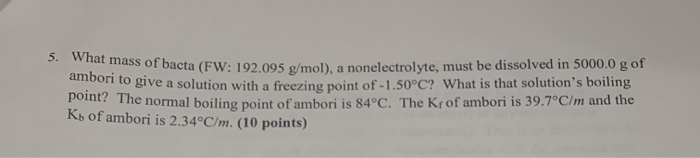thats all I know
5. What mass of bacta (FW: 192.095 g/m ambori to give a solution with a freezing po of bacta (FW: 192.095 g/mol), a nonelectrolyte, must be dissolved in 5000.0 g of "give a solution with a freezing point of -1.50°C? What is that solution's boiling the normal boiling point of ambori is 84°C. The Krof ambori is 39.7°C/m and the Ko of ambori is 2.34°C/m. (10 points)

#### Homework Answers

Answer #1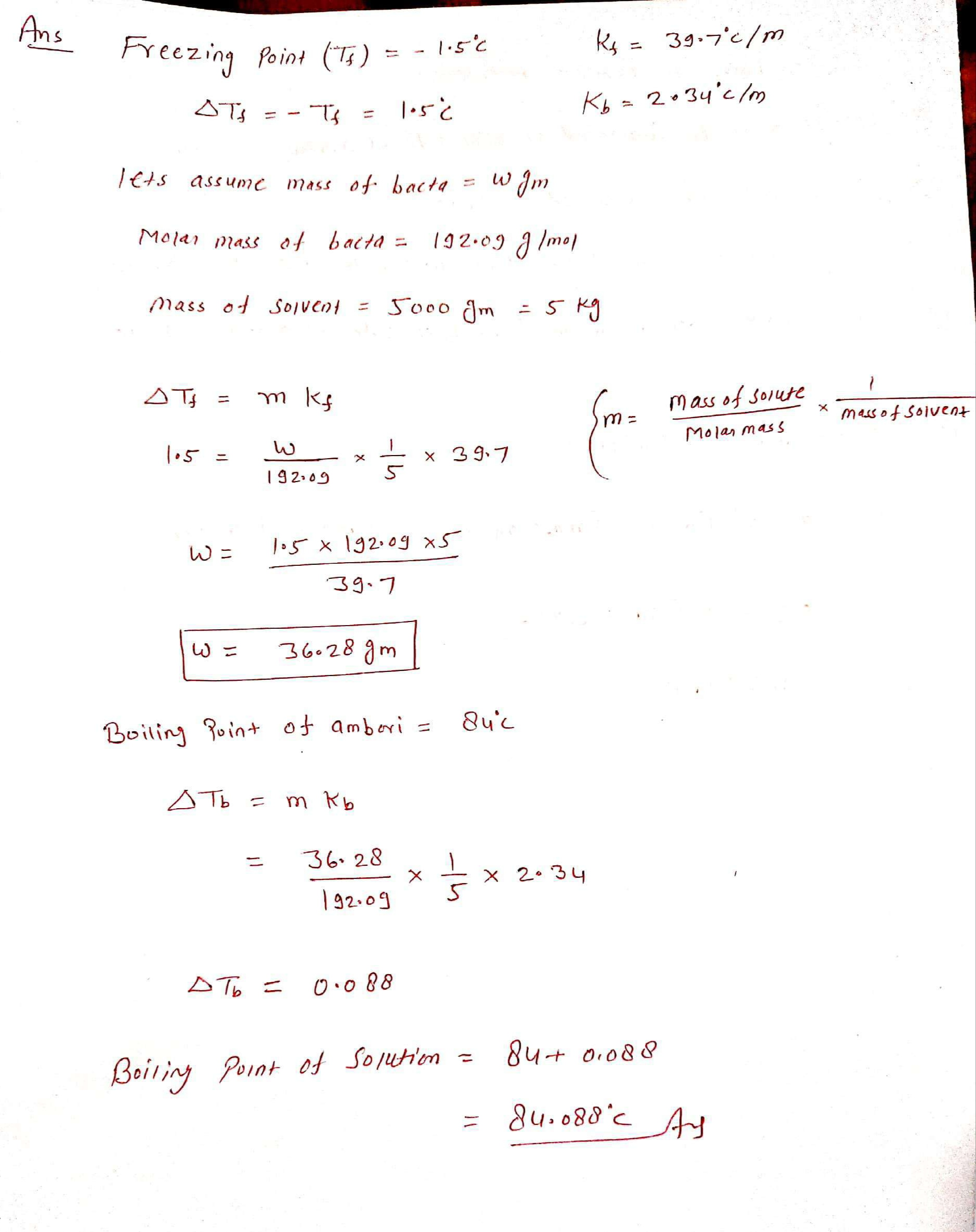Know the answer?
Your Answer:

#### Post as a guest

Your Name:

What's your source?

#### Earn Coin

Coins can be redeemed for fabulous gifts.

Not the answer you're looking for? Ask your own homework help question. Our experts will answer your question WITHIN MINUTES for Free.
Similar Homework Help Questions
• ### 5. What mass of bacta (FW: 192.095 g/mol), a nonelectrolyte, must be dissolved in 5000.0 g...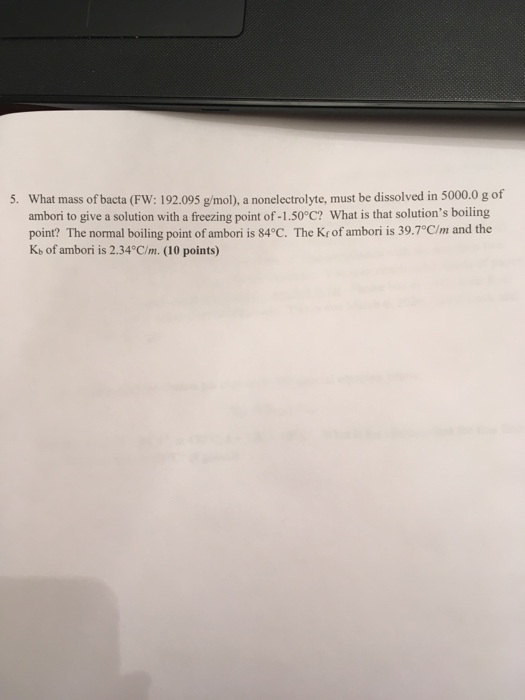5. What mass of bacta (FW: 192.095 g/mol), a nonelectrolyte, must be dissolved in 5000.0 g of ambori to give a solution with a freezing point of -1.50°C? What is that solution's boiling point? The normal boiling point of ambori is 84°C. The K of ambori is 39.7°C/m and the Ko of ambori is 2.34°C/m. (10 points)

• ### A solution is made by dissolving 0.745 mol of nonelectrolyte solute in 853 g of benzene....

A solution is made by dissolving 0.745 mol of nonelectrolyte solute in 853 g of benzene. Calculate the freezing point and boiling point of the solution. The normal freezing point of benzene is 5.49 °C and the normal boiling point of benzene is 80.1 °C. Assuming 100% dissociation, calculate the freezing point and boiling point of 0.570 mol of AgNO3 in 1.00 kg of water.

• ### Lysozyme extracted from chicken egg white has a molar mass of 13,930 g/mol. Exactly 0.1 g...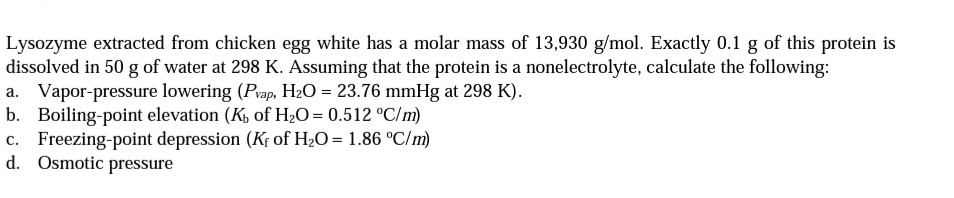Lysozyme extracted from chicken egg white has a molar mass of 13,930 g/mol. Exactly 0.1 g of this protein is dissolved in 50 g of water at 298 K. Assuming that the protein is a nonelectrolyte, calculate the following: a. Vapor-pressure lowering (Pvap, H20 = 23.76 mmHg at 298 K). b. Boiling-point elevation (Kb of H2O=0.512 °C/m) c. Freezing-point depression (Kf of H,O= 1.86 °C/m) d. Osmotic pressure

• ### 1.When a solution is made from 32.2 g of an unknown nonelectrolyte dissolved in 151 g...

1.When a solution is made from 32.2 g of an unknown nonelectrolyte dissolved in 151 g of solvent, the solution boils at 83.44 °C. The boiling point of the pure solvent and its Kb are 79.31 °C and 4.47 °C/m, respectively. Calculate the molar mass of the unknown electrolyte in g/mol. 2. Calculate the molar mass (in g/mol) of an unknown nonelectrolyte if 0.898 g dissolved in 268.7 mL of water at 30.13 °C has an osmotic pressure of 68.1...

• ### 8. What is the final boiling point of a 1.25 molal solution of sugar in water?...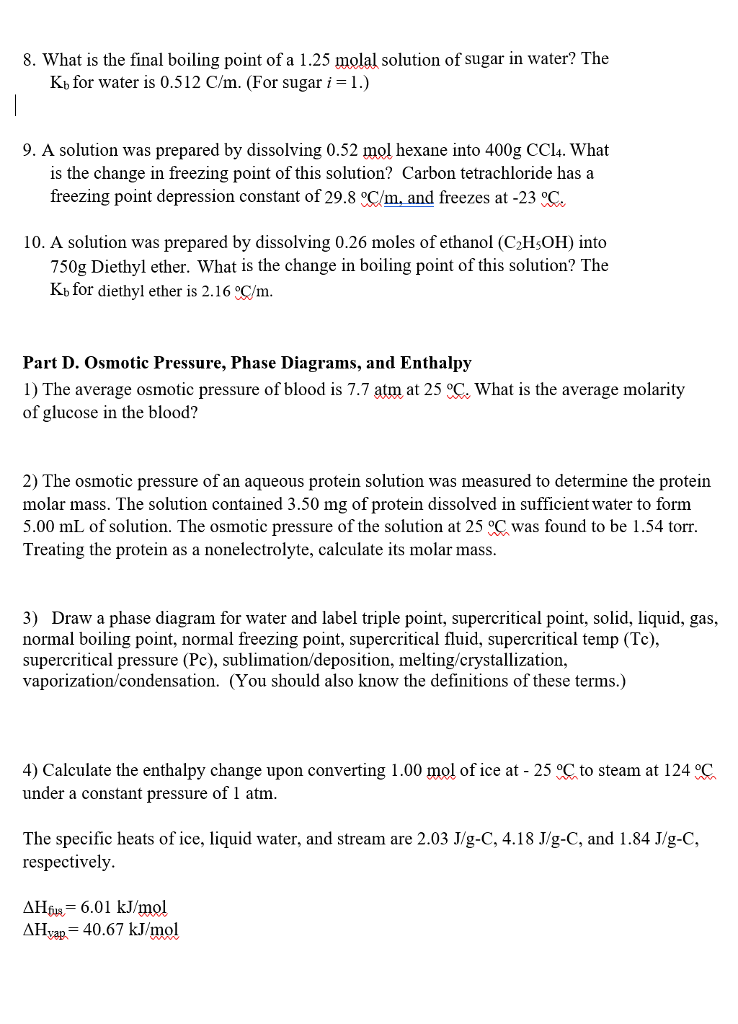8. What is the final boiling point of a 1.25 molal solution of sugar in water? The Kb for water is 0.512 C/m. (For sugar i = l.) 9. A solution was prepared by dissolving 0.52 mol hexane into 400g CCl4. What is the change in freezing point of this solution? Carbon tetrachloride has a freezing point depression constant of 29.8 Cm,and freezes at-23。. 10. A solution was prepared by dissolving 0.26 moles of ethanol (C2HsOH) into 750g Diethyl ether....

• ### What mass of glycerin (C,H,Os), a nonelectrolyte, must be dissolved in 200.0 g water to give a solution with a free...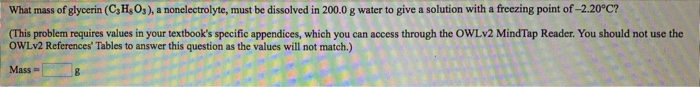What mass of glycerin (C,H,Os), a nonelectrolyte, must be dissolved in 200.0 g water to give a solution with a freezing point of -2.20°C? (This problem requires values in your textbook's specific appendices, which you can access through the OWLv2 MindTap Reader. You should not use the OWLV2 References' Tables to answer this question as the values will not match.)

• ### When 12.6-grams of an unknown compound is placed in 0.116-kg of benzene, the freezing point is...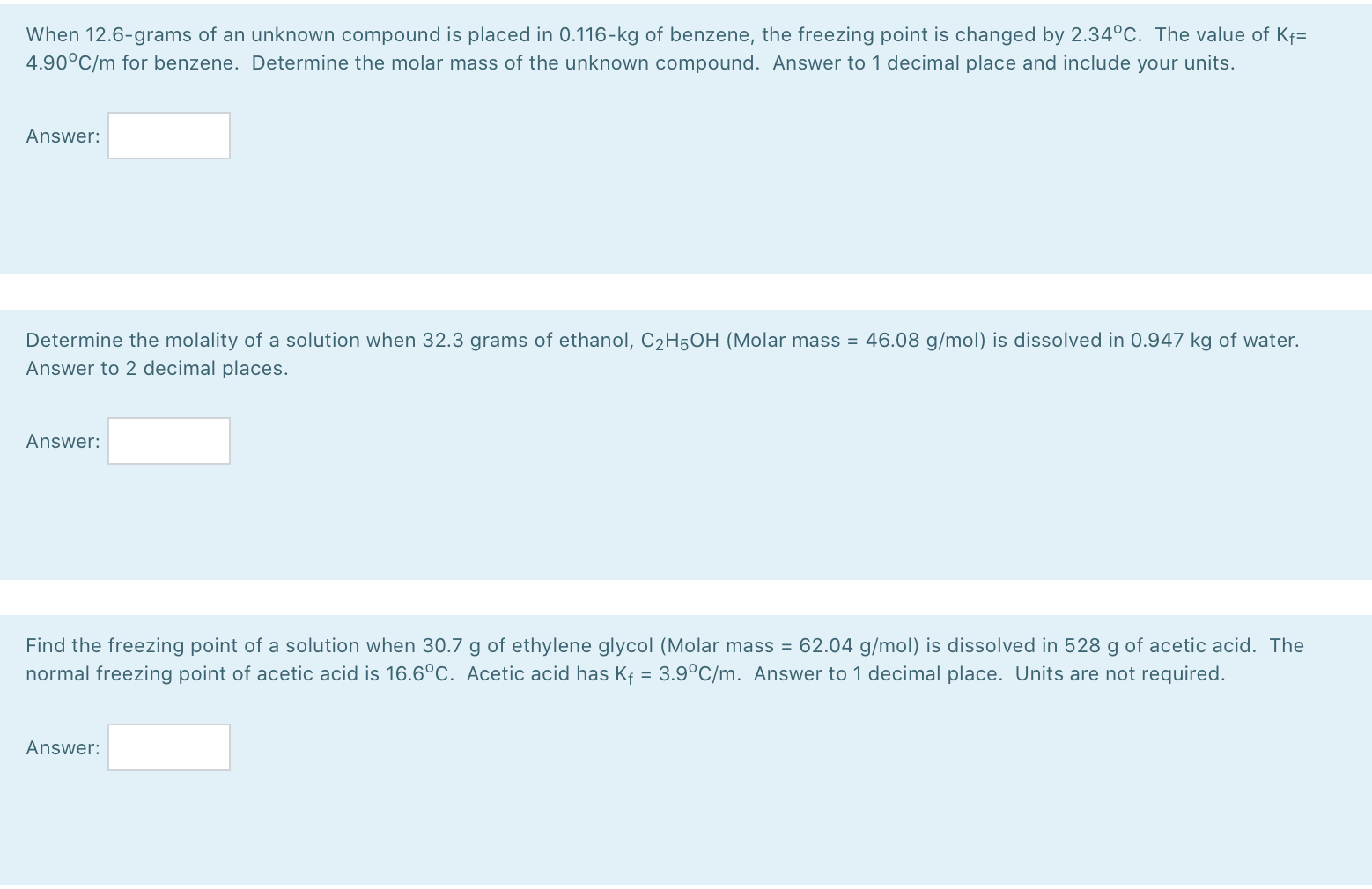When 12.6-grams of an unknown compound is placed in 0.116-kg of benzene, the freezing point is changed by 2.34°C. The value of Kf= 4.90°C/m for benzene. Determine the molar mass of the unknown compound. Answer to 1 decimal place and include your units. Answer: Determine the molality of a solution when 32.3 grams of ethanol, C2H5OH (Molar mass = 46.08 g/mol) is dissolved in 0.947 kg of water. Answer to 2 decimal places. Answer: Find the freezing point of a...

• ### A solution is made by dissolving 0.749 mol of nonelectrolyte solute in 861 g of benzene....

A solution is made by dissolving 0.749 mol of nonelectrolyte solute in 861 g of benzene. Calculate the freezing point, Tf, and boiling point, Tb, of the solution. Constants may be found here. Solvent Formula Kf value* (°C/m) Normal freezing point (°C) Kb value (°C/m) Normal boiling point (°C) water H2O 1.86 0.00 0.512 100.00 benzene C6H6 5.12 5.49 2.53 80.1 cyclohexane C6H12 20.8 6.59 2.92 80.7 ethanol C2H6O 1.99 –117.3 1.22 78.4 carbon tetrachloride   CCl4 29.8 –22.9 5.03 76.8...

• ### 5. When 4.50 g of a substance are dissolved in 125 g of CCl, (M-153.8 g/mol),...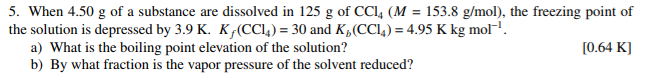5. When 4.50 g of a substance are dissolved in 125 g of CCl, (M-153.8 g/mol), the freezing point of the solution is depressed by 3.9 K. KCCl) 30 and K,(CC4) 4.95 K kg mol 0.64 K] a) What is the boiling point elevation of the solution? b) By what fraction is the vapor pressure of the solvent reduced? 1

• ### The boiling point of benzene CH is 80.10°C at 1 atmosphere. A nonvolatile, nonelectrolyte that dissolves...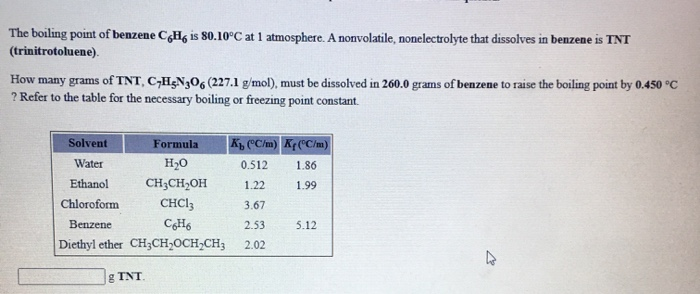The boiling point of benzene CH is 80.10°C at 1 atmosphere. A nonvolatile, nonelectrolyte that dissolves in benzene is TNT (trinitrotoluene) How many grams of TNT, C-H3N206 (227.1 g/mol), must be dissolved in 260.0 grams of benzene to raise the boiling point by 0.450°C ? Refer to the table for the necessary boiling or freezing point constant. Solvent Formula Ko (°C/m) Kr(°C/m) Water H20 0.512 1.86 Ethanol CH3CH2OH 1.22 1.99 Chloroform CHCI: 3.67 Benzene C6H6 2.53 5.12 Diethyl ether CH3CH...